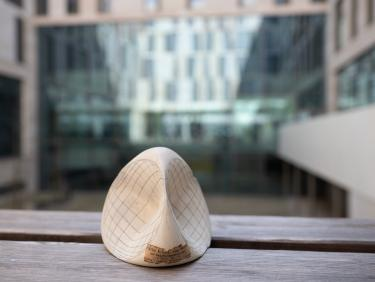# SectionAlgebra and Arithmetic Geometry

Arithmetic geometry is the study of rational solutions of systems of polynomial equations using the powerful machinery of algebraic geometry. Integer solutions of Diophantine equations are of particular importance to number theory, as are solutions in fields other than the rational numbers, such as finite fields.

Several motivating questions are more than 2000 years old, but only recent developments have made it possible to answer some of them. As a result, arithmetic geometry today is a vibrant, fascinating and very successful part of modern mathematics.

In Heidelberg, we focus on the following research areas. We study Galois representations and their deformations and the geometry of eigenvarieties over adic spaces as part of the p-adic Langlands program. Furthermore there is a field of focus on Drinfeld modular varieties as part of the Langlands program over function fields. We pursue research in Iwasawa theory, with a focus Iwasawa cohomology over p-adic Lie extensions with its relation to p-adic L-functions and p-adic Hodge theory. Furthermore there is a focus on arithmetic homotopy groups including the connection to Anabelian Geometry or the tame cohomology of adic spaces.## What is Arithmetic Algebraic Geometry about?

The classical objects of interest in arithmetic geometry are rational points, which are sets of solutions of a system of polynomial equations over number fields, finite fields, p-adic fields, or function fields. Of particular interest in number theory are integer solutions of Diophantine equations. Great progress was achieved by describing this algebraic setup in a geometric language and developing the powerful machinery of algebraic geometry. In particular, this led to a transformation of the initial problem to questions about the structure of algebraic varieties defined over non-algebraically-closed fields. This modern abstract version of algebraic geometry was developed by Grothendieck and Serre in the 1950/60s. Since then, arithmetic algebraic geometry has seen tremendous developments and successes. Prime examples are Deligne's solution of the Riemann Hypothesis for algebraic varieties over finite fields, Wiles' work on modularity implying Fermat's Last Theorem, and Lafforgue's contribution to the Langlands program. The speed of development in the field has kept pace, as the most recent list of Fields medals awarded in the area for Scholze and Venkatesh illustrates.

Typical overall research questions are

• How is the intrinsic geometry and arithmetic of spaces reflected by its cohomology, homotopy and Galois representations?
• How can algebraic structures that are governed by their monodromy or their towers of covering spaces be classified and computed?

## A Web of Methods and Conjectures in Arithmetic Geometry

Research in modern arithmetic geometry is integrated in a vast web of conjectures and uses a large toolbox of different methods.

Over finite fields, the theory of étale cohomology provides topological invariants associated to algebraic varieties. p-adic Hodge theory gives tools to examine when cohomological properties of varieties over the complex numbers extend to those over p-adic fields. The arithmetic of abelian varieties links the rational solutions (Mordell Weil group) with its Hasse-Weil L-function as conjectured by the (generalized) Birch and Swinnerton-Dyer conjecture, which in turn is a special case of the Tamagawa Number Conjecture. One way of studying these phenomena over towers of number fields simultaneously is by Iwasawa theory and p-adic L-functions, a vital research direction not least concerning possibly non-commutative equivariant aspects.

In a similar spirit but in a much more general framework the Langlands program, one of the largest research programs in pure mathematics, seeks to relate Galois groups and their representations to automorphic forms and the representation theory of algebraic groups over local and global fields – providing a vast generalization of class field theory. In particular it contains a correspondence of L-functions and local constants. One prominent recent generalization of Langlands’ classical conjectures is the p-adic Langlands program, where one studies the relationship of p-adic Galois representations to p-adic automorphic forms. Deformation techniques for Galois representations on the Galois side are paralleled by the study of geometric families of p-adic automorphic forms as given by eigenvarieties. Studying the interplay between the geometry of these families and the representation theory of the algebraic groups using cohomology theories is an interesting and highly active research direction. The question of how geometry dictates the image of Galois representations is the topic of another vibrant research direction. Deformation-theoretic arguments suggest that the image of the Galois representations is as large as geometry allows. These global aspects are complemented by the local p-adic Langlands program which is closely related to p-adic Hodge theory.

The occurrence of wild ramification in positive and mixed characteristic implies unexpected behaviour of classical geometric invariants. In particular, the higher étale homotopy groups of schemes over finite fields contain only limited information (they vanish for affine schemes). Therefore, there exists an enormous interest to develop the tame site of schemes and of adic spaces, a systematic way to avoid wild ramification. It will produce improved invariants, in particular homotopy-invariant cohomology and nontrivial higher homotopy groups.

Finally, anabelian geometry, which can be considered as another (highly non-linear and non-abelian) generalization of class field theory, describes the way in which the algebraic fundamental group π1(V,xˉ)π1​(V,xˉ) of a certain arithmetic variety V, or some related geometric object, can help to restore V.

Our research activities form part of the Collaborative Research Centre SFB 326 GAUS with partners in Frankfurt, Darmstadt, Mainz and Munich.

There has been a long tradition in arithmetic in Heidelberg with mathematicians like F.K. Schmidt, Maaß, Freitag, Roquette, Matzat, Wingberg et. al. In particular, the DFG- Research Groups “Arithmetic” (1998- 2004, Spokesperson: Freitag) and “Symmetry, Geometry, and Arithmetic (SGA)” (2013-2019, Spokesperson: Schmidt) have had a strong impact on the development of the field in Heidelberg.